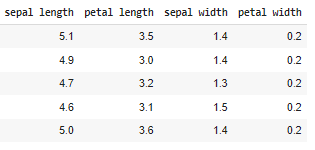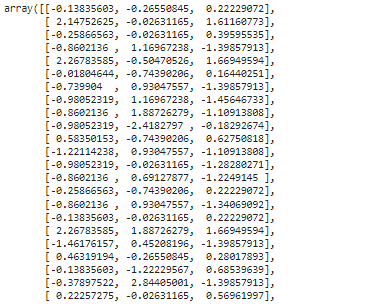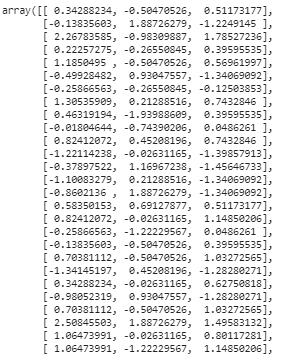GFG App
Open AppBrowser
Continue

# What is the difference between ‘transform’ and ‘fit_transform’ in sklearn-Python?

In this article, we will discuss the difference between ‘transform’ and ‘fit_transform’ in sklearn using Python.

In Data science and machine learning the methods like fit(), transform(), and fit_transform() provided by the scikit-learn package are one of the vital tools that are extensively used in data preprocessing and model fitting. The task here is to discuss what is the difference between fit(), transform, and fit_transform() and how they are implemented using in-built functions that come with this package.

• The fit(data) method is used to compute the mean and std dev for a given feature to be used further for scaling.
• The transform(data) method is used to perform scaling using mean and std dev calculated using the .fit() method.
• The fit_transform() method does both fits and transform.

All these 3 methods are closely related to each other. Before understanding them in detail, we will have to split the dataset into training and testing datasets in any typical machine learning problem. All the data processing steps performed on the training dataset apply to the testing dataset as well but in a slightly different format. This difference could be understood well when we understand these three methods.

Required Packages

`pip install scikit-learn`
`pip install pandas`

Let us consider we will have to perform scaling as one of the data processing steps to be performed. To demonstrate this example let us consider an inbuilt iris dataset.

Example:

## Python3

 `from` `sklearn ``import` `datasets ` `import` `pandas as pd ` ` `  `iris ``=` `datasets.load_iris() ` `data ``=` `pd.DataFrame(iris.get(``'data'``), columns``=``[ ` `    ``'sepal length'``, ``'petal length'``, ``'sepal width'``, ``'sepal width'``]) ` `data.head() `

Output:Iris dataset

Let us split the data as train and test splits.

## Python3

 `from` `sklearn.model_selection ``import` `train_test_split ` ` `  `X_train, X_test, y_train, y_test ``=` `train_test_split( ` `    ``data.iloc[:, :``-``1``], data[``'sepal width'``], ` `  ``test_size``=``0.33``, random_state``=``42``) `

Now let us perform a standard scaling on the sepal width column. Scaling in general means converting the column to a common number scale, Standard scaling in particular converts the column of interest by transforming it to a range of numbers with mean = 0 and standard deviation = 1.

## The fit() Method

The fit function computes the formulation to transform the column based on Standard scaling but doesn’t apply the actual transformation. The computation is stored as a fit object. The fit method doesn’t return anything.

Example:

## Python3

 `from` `sklearn.preprocessing ``import` `StandardScaler ` ` `  `scaler ``=` `StandardScaler() ` `scaler.fit(data[``'sepal width'``]) `

Output:

`StandardScaler()`

## The transform() Method

The transform method takes advantage of the fit object in the fit() method and applies the actual transformation onto the column. So, fit() and transform() is a two-step process that completes the transformation in the second step. Here, Unlike the fit() method the transform method returns the actually transformed array.

Example:

## Python3

 `scaler.transform(data[``'sepal width'``]) `

Output:Output of standard scaler

## The fit_transform() Method

As we discussed in the above section, fit() and transform() is a two-step process, which can be brought down to a one-shot process using the fit_transform method. When the fit_transform method is used, we can compute and apply the transformation in a single step.

Example:

## Python3

 `scaler.fit_transform(X_train) `

Output:Output of fit_transform

As we can see, the final output of fit(), transform(), and fit_transform() is going to be the same. Now, we will have to ensure that the same transformation is applied to the test dataset.  But, we cannot use the fit() method on the test dataset, because it will be the wrong approach as it could introduce bias to the testing dataset. So, let us try to use the transform() method directly on the test dataset.

Example:

## Python3

 `scaler.transform(X_test) `

Output:Transformation of test dataset

As we can see, both have different outputs this could be one of the reasons that sklearn has split this kind of data processing step into two.

My Personal Notes arrow_drop_up